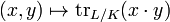# Different ideal

In algebraic number theory, the different ideal is an invariant attached to an extension of algebraic number fields.

Let L/K be such an extension, with rings of integers OL and OK respectively. The relative trace defines a bilinear form on L by$(x,y) \mapsto \mathrm{tr}_{L/K} (x \cdot y) \,$

in which the dual of OL is a fractional ideal of L containing OL. The (relative) different δL/K is the inverse of this fractional ideal: it is an ideal of OL.

The relative norm of the relative different is equal to the relative discriminant ΔL/K. In a tower of fields L/K/F the relative differents are related by δL/F = δL/K δK/F.

## Ramification

The relative different encodes the ramification data of the field extension L/K. A prime ideal p of K ramifies in L if and only if it divides the relative discriminant ΔL/K. If

p = P1e(1) ... Pke(k)

is the factorisation of p into prime ideals of L then Pi divides the relative different δL/K if and only if Pi is ramified, that is, if and only if the ramification index e(i) is greater than 1. The precise exponent to which a ramified prime P divides δ is termed the differential exponent of P and is equal to e-1 if P is tamely ramified: that is, when P does not divide e. In the case when P is wildly ramified the differential exponent lies in the range e to eP(e)-1.

## Local computation

The different may be defined for an extension of local fields L/K. In this case we may take the extension to be simple, generated by a primitive element α which also generates a power integral basis. If f is the minimal polynomial for α then the different is generated by f'(α).Some content on this page may previously have appeared on Citizendium.